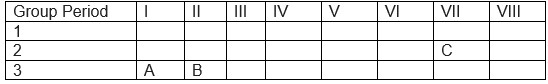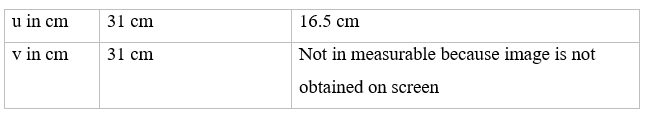# Class 10 Science Half Yearly Sample Paper -2 | TERM 1

In this page we have Class 10 Science Half Yearly Sample Paper -2 . Hope you like them and do not forget to like , social share and comment at the end of the page.
Section-A
Question 1.
Write name of the compound: CH3-CH2-CHO

Question 2.
Trilobite, Ammonite is which type of fossils?

Question 3.
Define biological magnification.

Question 4.
Illustrate why there should be equitable distribution of resources?

Question 5.
Suggest some approaches that need to be adopted towards conservation of forest.

Question 6.
A person suffering from an eye-defect uses lenses of power 1D to correct the defect. Name the defect he is suffering from and the nature of lens to be used to correct it.

Question 7.
Explain the changes that occur in ovary and an ovule after fertilization in a flower

Question 8.
The position of three elements A, B and C in the periodic.
Table is shown below:Given reasons, explain
(a) Element A is mental. (b) Element B has larger atomic size than the element C.
(c) Element C has a valenmcy-1

Question 9.
Two elements X and Y have atomic number 11 and 17 respectively.
(a) Write the electronic configuration of both
(b) Which type of bond will they form?
(c) Write the formula of the compound formed by them with each other

Question 10.
Write balanced chemical equation of the reaction of ethanoic acid with:
(i) Sodium carbonate (ii) Sodium hydrogen carbonate
(iii) Sodium hydroxide

Question 11.
(a) Identify the group of the metal (M), the formula of whose Chloride is:
(i) MCP (ii) MC
(b) Identify the group of the nonmetal (X), the formula of whose sodium salt is:
(i) Na2X (ii) Nax
(c) Write the electronic configuration of Argon.

Question 12.
Explain the process of regeneration as seen in Planaria.

Question 13.
How do species of two isolated subpopulations become two different species?

Question 14.
Guinea-Pig having black colour when crossed with guinea-Pig having some colour, produced 80 off springs out of this 60 were black and 20 were black.

Question 15.
What is the genotype of guinea-pig?

Question 16.
Which is a dominate and which a restraint trait?

Question 17.
What is this type of cross called as?

Question 18.
(a) How would you relate the following methods to asexual reproduction?
(i) Binary fission (ii) Spore formation
(b) State any advantage of using sexual reproduction over asexual reproduction.

Question 19.
A convex lens produces an image on screen twice the size of the object. The distance between the screen and the object is 45 cm. Find the distance of the lens from the object and focal length of the lens.

Question 20.
Explain in brief the Tyndall effect. State the reason behind:

(a) The blue colour of sky (b) Red colour for danger signals

Question 21.
(a) Define magnification by a spherical mirror and express it in Terms of object distance and image distance for the mirror.
(b) The magnification produced by a convex lens is-2. What is meant by this statement and also write the information regarding image obtained form it?

Question 22.
Mineral riches of the crust are either extracted or used. For every 3 ton of metal a large amount of slag is discarded which damages the environment. Explain the kind of management that we need in this regard. Give any two valued attained from this management system.

Question 23.
(a) Describe an activity to show action of soap and detergent on hard water.
(b) Name the substances where chemical salts are present in;
(i) Detergent (ii) hear water (iii) soap

Question 24.
Wings of bats and birds have different design.
(a) Explain in which way they are different.
(b) In which aspect do the wings of bat and bird resemble?

Question 25.
(a) Give appropriate terms for the following.
(i) Trait which expresses itself in next generation.
(ii) The trait an organism has due to inheritance.
(iii) Origin of new species from existing ones.
(b) Genes are the unit of inheritance. Mention any two characteristic of genes.

Question 26.
(a) Define dispersion of light. (b)How is scattering of light different form dispersion? Give one example of natural phenomenon based on each of these.

## Section-B

### Multiple Choice Questions

Question 27.
The odour of Ethanoic acid resembles with:
(i) Tomato Juice
(ii) Kerosene
(iii) Orange Juice
(iv) Vinegar

Question 28.
A burning splinter is introduced near the mouth of four test tubes while adding acetic acid to the chemical present in them. The splinter will get extinguished in the following case.
(i) Sodium chloride+ acetic acid
(ii) Sodium hydroxide+ acetic acid
(iii) Sodium bicarbonate+ acetic acid
(iv) Sodium metal+ acetic acid

Question 29.
During saponification, along with soap, another compound is also formed. The compound is:
(a) Glycol
(b) Glycogen
(c) Glycerol
(d) Glucose

Question 29.
The preparation of soap is also known as:
(a) Etherification
(b) Condensation
(c) Saponification
(d) Oxidation

Question 30.
Soap solution in water is
(a) Clear salutation
(b) Homogeneous solution
(c) Colloid solution
(d) Suspension

Question 31.
A student obtained a sharp image of a near object on a screen using a convex lens. For getting better results, the teacher suggested focusing of a distant building instead of the of the object. In which direction should the lens be moved for this purpose?

(a) Away from the screen
(b) Behind the screen
(c) Very far away from the screen
(d) towards the screen

Question 31.
In the experiment on refraction of light through a glass slab done by four students A, B, C and D, the following observations were made:
(A) The emergent ray moves towards the normal after second refraction through glass slab with i= e
(B) The emergent ray moves away from the normal after second refraction through glass slab with l<= e
(C) For any angle of incidence, al; ways l= e
(D) The emergent ray moves wasy from normal after second refraction through glass slab with i= e
The student who has made the correct observation is:
(a) (A)
(b) (B)
(c) (C)
(d) (D)

Question 32.
After students had observed the slides of binary fission of amoeba, a student was asked by his teacher why this process was called binary fission. Out of the four statements given by different students, which statements are correct?
(a) One parent divides into two daughter cells.
(b) Two daughter cells combine to form a new cell.
(c) One parent and one daughter cell involved in the process.
(d) Two parent cells combine to form daughter cells.

Question 33.
The following events explain budding in years:-
(P) Bud remains attached to the parent body and gives rise to new bud.
(Q) The process may be repeated 3-4 times and a chain of buds is formed
(R) The bud upon maturity gets separated from the parent body and give rise to new bud
(S) Bulb- Like projection is given out from the body of yeast, the nucleus divides into two and one of the daughter nuclei moves in the bud.
Identify the correct sequence of events.
(i) (P), (S), (Q), (R)
(ii) (S), (R), (P), (Q)
(iii) (S), (P), (Q), (R)
(iv) (R), (S), (P), (Q)

Question 34.
When a ray of light enters from air to the rectangular glass prism then it emerges out of the prism. In these situations the relation between the angle of incidence and angle of emergence is:
(a) Angle of incidence is smaller than the angle of emergence
(b) Angle of incidence is larger than the angle of emergence
(c) Angle of incidence is equal to angle of emergence
(d) None of the above.

Question 35.
A rectangular glass prism has:
(a) two triangular bases and three rectangular lateral surfaces.
(b) Two triangular bases and two rectangular lateral surfaces.
(c) Two triangular bases and four rectangular lateral bases.
(e) Three triangular bases and two rectangular lateral surfaces.

Question 36.
A student was doing an experiment to check the focal length of a converging lens whose focal length as marked on its cover was 15.5 cm. he then placed an object at different positions in front of the lens and tried to get image on a screed. The table shows the measurements recorded by the student for the object distance u and the image distance vHe came to the conclusion that
(a) The focal length of lens is same as mentioned
(b) Focal length was lesser than mentioned.
(c) The focal length of lens is larger than mentioned.
(d) The focal length of lens cannot be estimated.

Question 37.
While doing an experiment it convex lens a student found that the image distance of object is equal to object ideate and also the heights of object and the image are equal height, in this situation the position of object is at:
(a) Focus of lens
(b) at double the focal length.
(c) Between focus and optical center
(d) Between focus and center of curvature.
Question 38.
Wings of a bird, a bat and a housefly are…………to each other
(a) Homologous
(b) vestigial
(c) analogous
(d) Rudimentary

Question 39.
The future foot present on embryonic asis in the seed is called:
(a) Cotyledon
(c) Plumule
(d) Hypocoty1

## Summary

This Class 10 Science Half Yearly Sample Paper -2 for TERM 1 is prepared keeping in mind the latest syllabus of CBSE . This has been designed in a way to improve the academic performance of the students. If you find mistakes , please do provide the feedback on the mail.